# SSC CGL EXAMS 2019 | Quantitative Aptitude Practice Questions (Day-21)

Dear Aspirants, Here we have given the Important SSC Exam 2018 Practice Test Papers. Candidates those who are preparing for SSC 2019 can practice these questions to get more confidence to Crack SSC 2019 Examination.

[WpProQuiz 4852]

1) A deer running on a region of the forest where the shape of the region is triangular starts running from point B to A, then A to C and finally reach on point B. If the speed of deer is 120 km/h, in what time deer complete his journey. Some more information regarding the journey of deer ‘is mentioned below’.

i) Deer turn right on Point A with 1000

ii) The turning angle at C is 200 rightward.

iii) The distance between B to C is 100 km & B to A is 80 km.

a) (9 + √21)/6 h

b) (9 − √21)/6 h

c) (9 ± √21)/6 h

d) 30 h

2) If a, b, c, d, e & f are six different positive integers selected from 1 to 35, then the highest possible value of would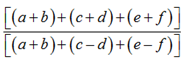be.

a) 80

b) 70

c) 79

d) 75

3) Two diagonals of a parallelogram intersect each other at coordinates (7.75, 13.75). Two adjacent points of the parallelogram is (3.15, 5.75) and (11.75, 14). Find the approximate lengths of the diagonals?

a) 4

b) 6

c) 8

d) 5

4) A man borrows Rs. 12, 000 at 10% interest, on decreasing balance, at the start of the year. If he repays 2400 at the end of each year, find the amount of loan outstanding, (Rs) at the beginning of the third year.

a) Rs. 10800

b) Rs. 10480

c) Rs. 9000

d) Rs. 9480

5) If cot A. cot B = 4, then what is the value of 3cos (A + B) + sec (A – B)?

a) 9 1/5

b) 8 1/5

c) 7 1/5

d) 6 1/5

6) What is the minimum value of y = sin12θ+ cos10 θ + 2sin8 θ + 3cos6 θ?

a) 64/43

b) 43/64

c) 0

d) 1

7) Bean has purchased a van for Rs.12000/, he sells it to Dayal on alacs of 20%, Again Dayal sells it to Amit on profit of 30%, lastly, Amit sells it to Vinita on 10% loss, then what is the actual cost paid boy Vinita to Amit?

a) Rs.112320

b) Rs.110000

c) Rs. 120000

d) None of these

8) Find the difference between areas of the given figures?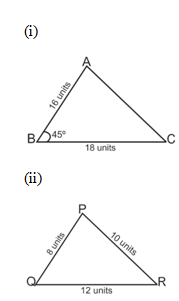a) 87 √2 unit2

b) 72 √2 unit2

c) 15 √2 unit2

d) 57 √2 unit2
9) What is the successive discount of 10%, 20%, 30% and 40%?

a) 50%

b) 65%

c) 69.76%

d) 70%

10) The average weight of 18 tall boys is a class is 48.03 kg and that of the remaining 7 boy is 40.05 kg. Find the average weight of all boys is the class

a) 40.7056

b) 50

c) 35.5

d) 45.7956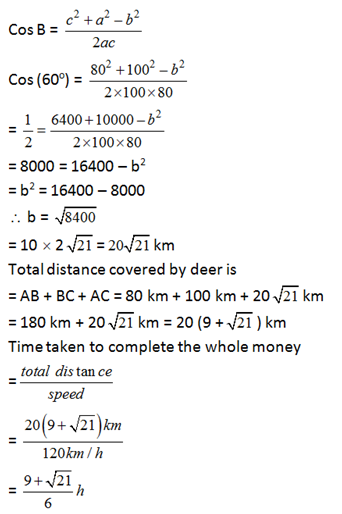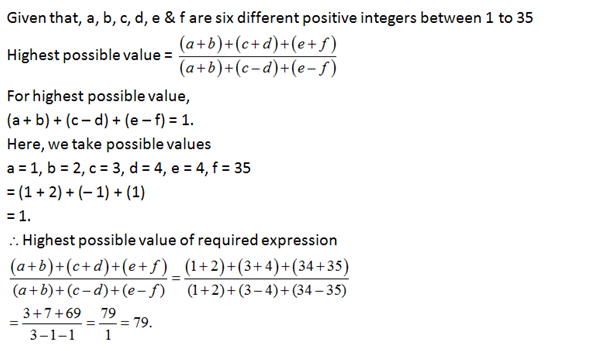Since diagonals of the parallelogram intersect each other at mid-points.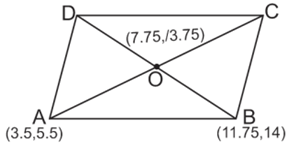Distance between points (7.75, 13.75) and (3.75, 5.75),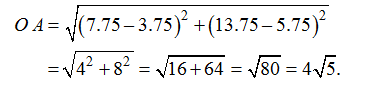Distance between points (7.75, 13.75) & (11.75, 14)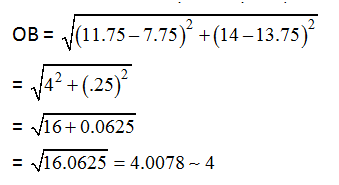After one year, amount due

12000×1.1 – 2400 = 10,800

So, amount due after two years,

= 10,800×1.1 – 2400

= Rs.9480

Given that, cot A. cot B = 4   ……… (i)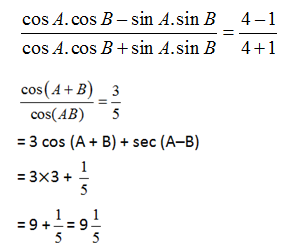If  Y is minimum, then θ should be 450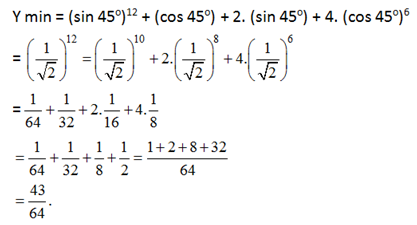According to questions,

Actual cost paid by Vinita to Amit is,

= 120000 * (80/100) * (130/100)* (90/100) = 112320

Area of the (1) figure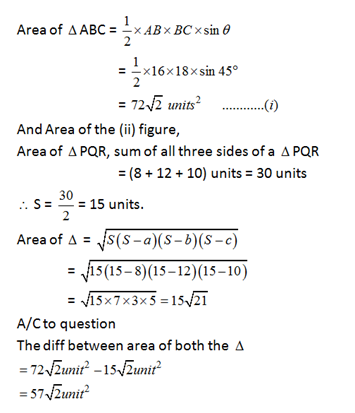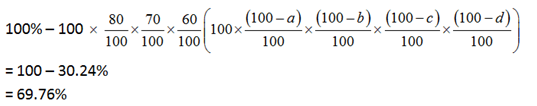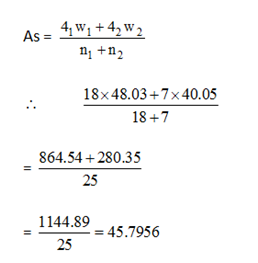### *******************

#### SSC CGL EXAMS 2019 |Quantitative Aptitude Practice Questions (Day-18)